# Implementing Pairs Trading/Statistical Arbitrage Strategy In FX Markets [EPAT PROJECT]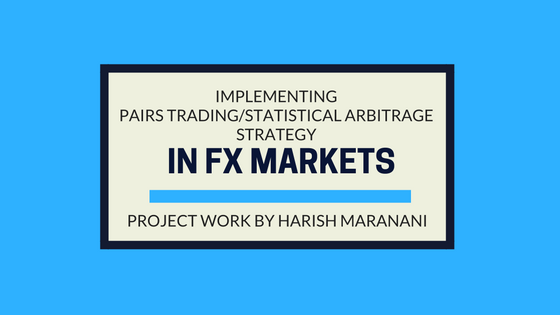This article is the final project submitted by the author as a part of his coursework in Executive Programme in Algorithmic Trading (EPAT™) at QuantInsti. Do check our Projects page and have a look at what our students are building.Harish Maranani is an EPATian. His educational qualifications include:

• Bachelors in Technology in Electronics and Communications Engineering from Acharya Nagarjuna University,
• MBA Finance from Staffordshire University (UK),
• Certificate in Quantitative Finance (CQF), and
• Master of Science in Mathematical and Computational Finance from New Jersey Institute of Technology, Newark, USA.

Harish was enrolled in the 27th Batch of EPAT™, and this report is part of his final project work.

Aim: To implement pairs trading/statistical arbitrage strategy in currencies.

Pairs Chosen: EURINR, USDINR, GBPINR, AUDINR, CADINR, JPYINR

Frequency: Daily

Time Period: 2011/4/21 to 2013/5/22

Implemented using: Python.

Pair Selection Criteria for FX Markets:

• The time series data for the above-chosen currency pairs is imported from quandl.
• Co-integration Test is carried out on all possible pair combinations viz. EURINR-USDINR, EURINR-GBPINR etc.
• Selecting Co-integrated pairs whose t-static value is less than 5% critical value of -2.8.
• Slicing the pairs which meet the co-integration condition for further analysis.
• To further test for confirmation of co-integration, CADF test is carried out on the sliced pairs from the pool.
• Z-score is calculated for each selected pair combination and the strategy is applied.
• Profit/loss, equity curve, maximum drawdown, are calculated/tabulated/plotted.
• Consider two currency pairs EUR/INR and USD/INR. Here the base currencies are EUR and USD respectively and the counter currency is INR.

Preliminary Test:

• In order to find the pairs of currencies that are co-integrated, a preliminary test through coint(x,y) from statsmodels.tsa.stattools is carried out and their respective pvalues, tstatic are plotted below.
• The t-static values that are displayed below are the ones that passed the co-integration test. i.e the t-static values smaller than the 5% critical value of -2.86.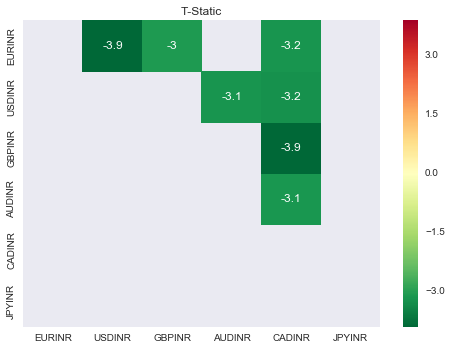Below is the list of pairs whose T-static values are less than the 5% critical value of -2.86:

• ['EURINR/USDINR: -3.89372142826',
• 'EURINR/GBPINR: -3.04457063111',
• 'USDINR/AUDINR: -3.14784526027',

Below is the plot of p-values of the co-integrated pairs: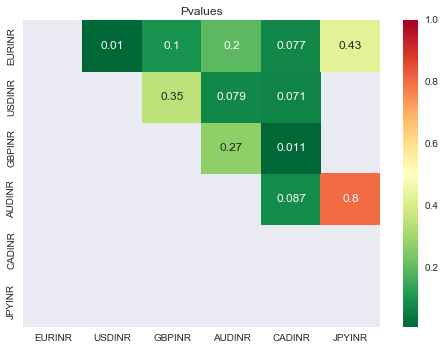Before rejecting null hypothesis to confirm the prices are mean-reverting, we shall conduct Co-Integrated Augmented Dickey-Fuller (CADF) test to confirm the same for the above sliced pairs out from the whole set of currencies. Below are the Results and plots.

We shall consider the 4 co-integrated pairs based on T-Static Values for CADF testing.

The following are the 4 Co-integrated pairs:

EURINR/USDINR:  -3.89372142826

#### EURINR/USDINR

TIME SERIES PLOTS OF EURINR/USDINR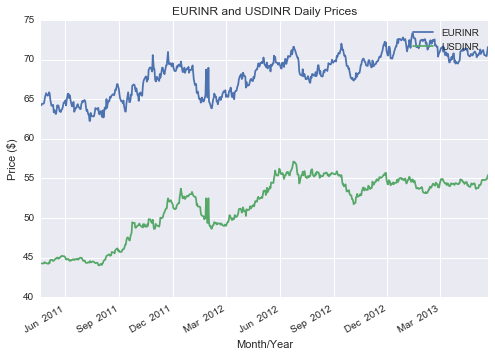From the above graph, it is visibly evident that the prices are co-integrated, however, to statistically confirm the same, the below set of tests/procedures are implemented.

####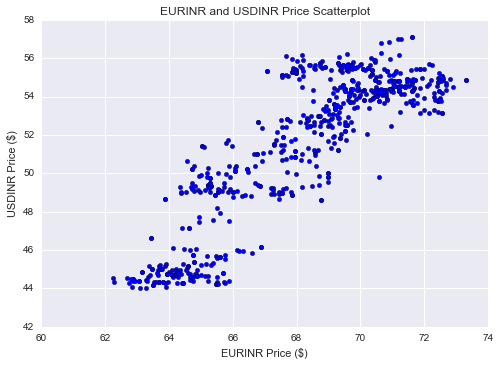Creating a scatter plot of the prices, to see the relationship is broadly linear.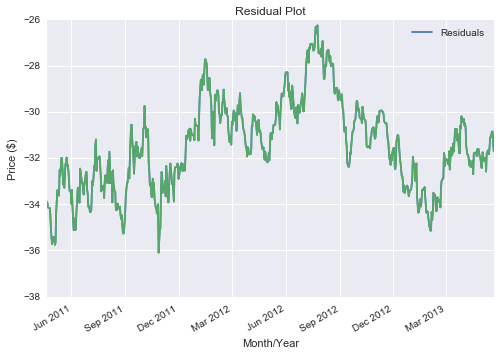Given the above residual plot, it is relatively stationary.

##### Co-integrated Augmented Dickey-Fuller Test Results

Co-integrated Augmented Dickey-Fuller (CADF) test determines the optimal hedge ratio by performing a linear regression against the two-time series and then tests for stationarity under the linear combination.

Implementing in python gives the following result:

(-3.0420602182962395,

0.03114885626164075,

1L,

652L,

{'1%': -3.440419374623044,

'10%': -2.5691361169972526,

'5%': -2.8659830798370352},

852.99818965061797)

Given the above results, the t-static to be -3.04 less than 5% critical value of -2.8, we can reject the null hypothesis and can confirm that the prices are mean-reverting.

Below are the time series, scatter and residual plots of GBPINR/CADINR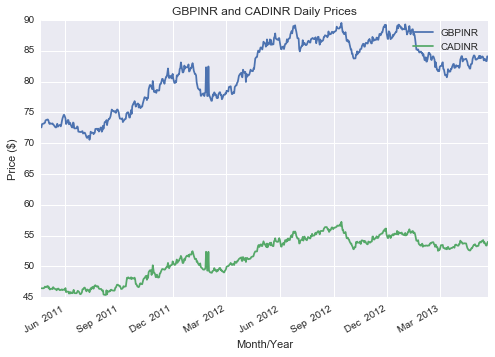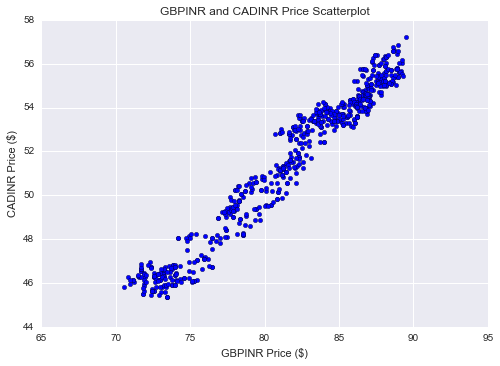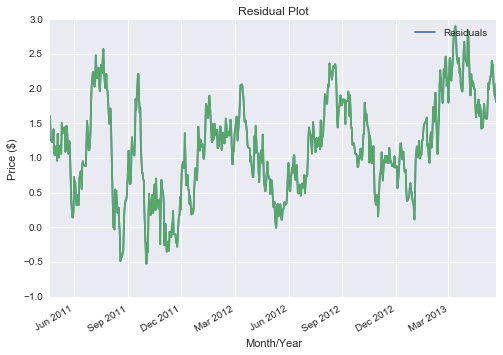(-3.3637522231183872,

0.012258395060108089,

2L,

651L,

{'1%': -3.440434903803665,

'10%': -2.569139761751388,

'5%': -2.865989920612213},

-179.04749802146216)

Given the above results, the t-static to be -3.36 smaller than 5% critical value of -2.8, we can reject the null hypothesis and can confirm that the prices are mean-reverting.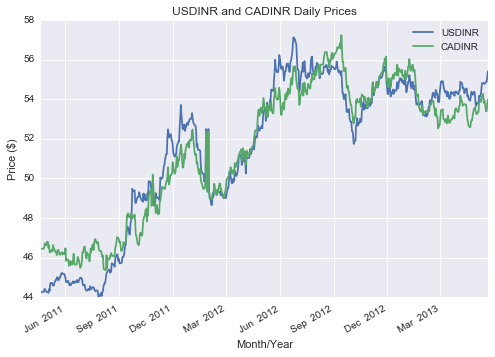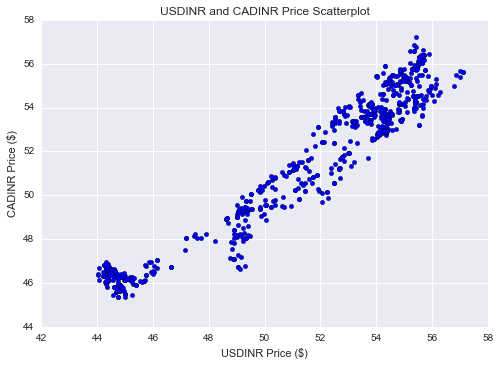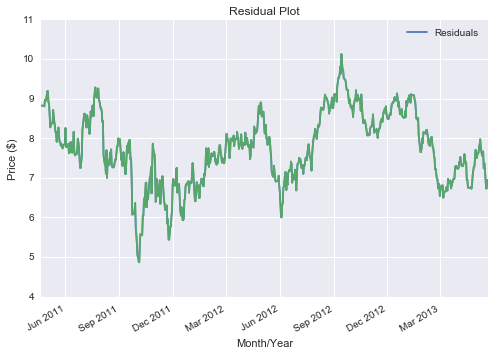Given the above results, the t-static to be -2.93 smaller than 5% critical value of -2.8, we can reject the null hypothesis and can confirm that the prices are mean-reverting.

(-2.9344605252608607,

0.041484961304201866,

1L,

652L,

{'1%': -3.440419374623044,

'10%': -2.5691361169972526,

'5%': -2.8659830798370352},

-99.577663481220952)

#### USDINR/AUDINR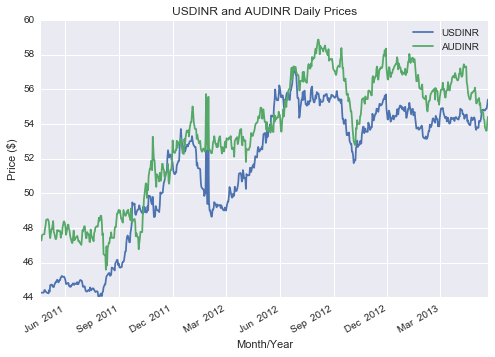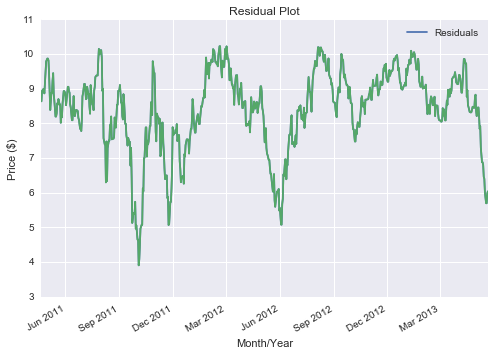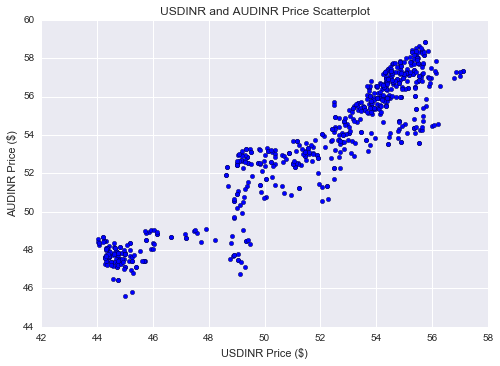Below are the results from CADF test:

(-3.2595055880757768,
0.016788501512565262,
4L,
649L,
{'1%': -3.440466106307706,
'10%': -2.5691470850496558,
'5%': -2.8660036655537744},
381.77145926378489)

With the t-static value of -3.25 smaller than the 5% critical value of -2.86, we can reject the null hypothesis and can confirm that the pair is co-integrating.

Now that we have found the co-integrated pairs in the form of following pairs with t-static values:

• EURINR/USDINR: -3.04
• USDINR/AUDINR: -3.259

Next Step would be to calculate the Z-score of the price ratio for 30day moving average and 30day standard deviation:

• Calculating price ratios and creating a new column ratio in the data frames (df, df1, df2, df4) of the above currency pairs respectively.

Below is the snapshot of the data frames:

df: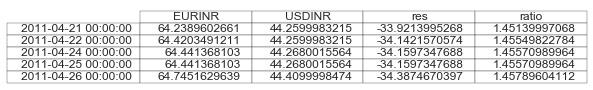Df1: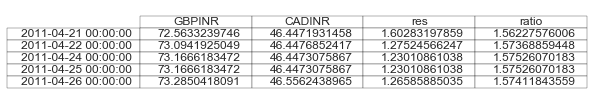Calculation of Z-score of the price ratio for the 30-day window of moving average and standard deviation:

• Below are the plots of z-scores for the above co-integrated pairs with their respective price ratios: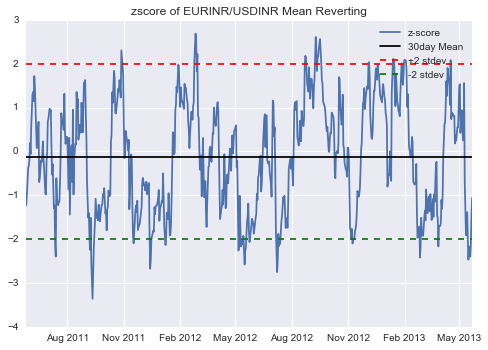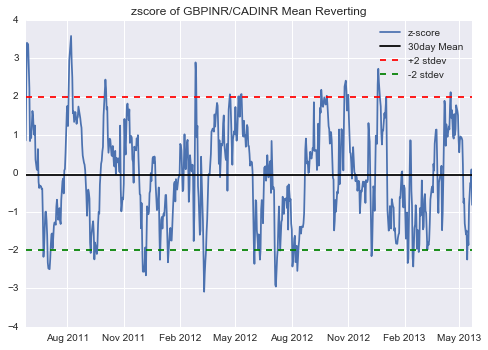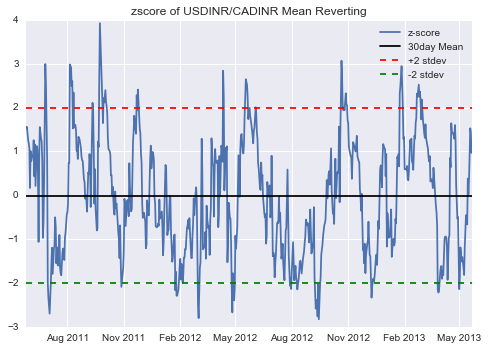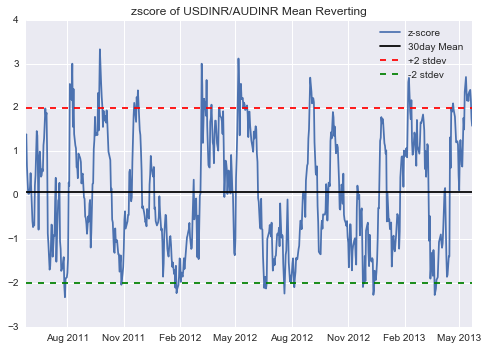From the above Z-Score plots of the selected pairs, Z-score is exhibiting mean reverting behavior within 2 standard deviations.

• When z-score touches +2 short the pair and close the position when it reverts back to +1
• When z-score touches -2 long the pair and close the position when it reverts back to -1.
• Only one position is held at a single instance of time.

Equity Curve:

Plotting the equity curve with the starting capital of 100 INR equally divided among 4 pairs.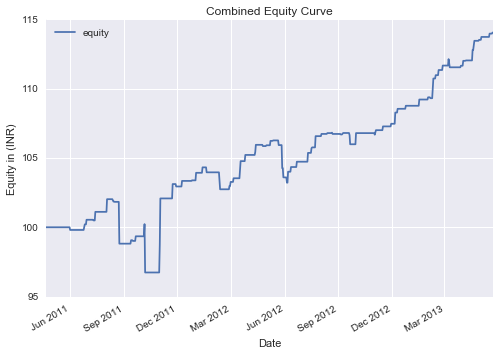With 100 INR initial Capital, equity ended at 114.05.

Cumulative profit to be 14% without any leverage. With 10 times leverage (ideal for FX trading), the profits can be seen at 140%. Below are the important performance metrics of the strategy.

 Profit percentage Without Leverage 14.0514144897 % Profit percentage with 10 times leverage 140.514144897 % Number of Positive Trades 59 Number of Negative Trades 23 Hit Ratio 71.9512195122 % Average Positive Trade 0.46886657456220338 Average Negative Trade -0.59181362649660851 Average Profit/Average Loss 0.792253766338 Maximum Drawdown -5.1832506579 %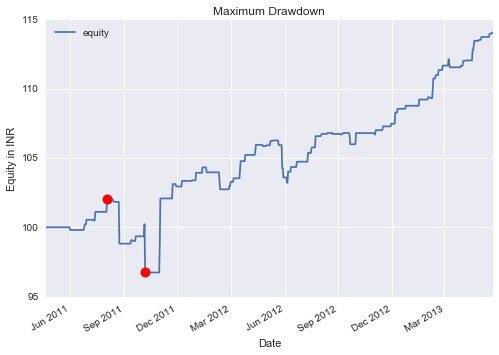The above graph shows the maximum drawdown points marked with red dots and the value is added in the above table.

### Instructions for Implementation

• Please run the IPython notebook named harish_stat_arb.ipynb for the confirmation of results and plots.
• Another option is to run the python script harish_quantinsti_final_project_code.py on any python IDE to confirm the results and graph.
• Use the below code for exporting the final dataframe to an excel file.
writer = pd.ExcelWriter('pairs_final.xlsx',engine = 'xlsxwriter')

pairs.to_excel(writer,'Sheet5')

writer.save()

### Conclusion

Though the strategy has generated 140% returns over the backtest period of 2 years, the following factors should be considered in order to evaluate a more accurate performance of the strategy.

• The model has ignored the slippage and commissions